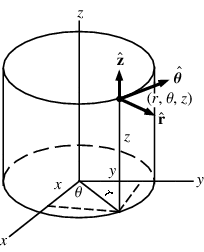# Electric Field of a ring , mathematically

• MinaKaiser
This means that at any other point (r', theta', z') we have a different basis for \hat r.We can use this fact to find the equation for \hat r:\hat r = \hat x \cos \theta + \hat y \sin \thetaf

#### MinaKaiser

This is about the electric field of a ring with radius r, at a distance z from center, along the axis of the ring. The ring carries a uniform line charge
λ
. We always say that the radial component of the field cancels out due to symmetry. Can somebody tell how to prove it mathematically (using cylindrical coordinate system only)?

Welcome to PF, MinaKaiser!This is about the electric field of a ring with radius r, at a distance z from center, along the axis of the ring. The ring carries a uniform line charge
λ
. We always say that the radial component of the field cancels out due to symmetry. Can somebody tell how to prove it mathematically (using cylindrical coordinate system only)?

First, let's clean up your formula.

I get:

$$d\vec E_{rad} = - {1 \over 4\pi \epsilon_o} \cdot {\lambda r d\theta \over r^2 + z^2} \cdot {r \over \sqrt{r^2 + z^2}} \hat r$$

$$\vec E_{rad} = - {1 \over 4\pi \epsilon_o} \cdot {\lambda r d\theta \over r^2 + z^2}\cdot {r \over \sqrt{r^2 + z^2}} \int_0^{2\pi} \hat r d\theta$$

So what we need is:
$$\int_0^{2\pi} \hat r d\theta = 0$$

Since $\hat r$ is not a constant vector, we rewrite it in a basis that is constant:

$$\hat r = \hat x \cos \theta + \hat y \sin \theta$$

So
$$\int_0^{2\pi} \hat r d\theta = \int_0^{2\pi} (\hat x \cos \theta + \hat y \sin \theta) d\theta$$

Can you see that this is zero?

I dont' get it , Why r is not a constant vector ?

I dont' get it , Why r is not a constant vector ?

Here's a picture of cylindrical coordinates:At some point (r, theta, z) we have a local basis which contains $\hat r$.
But if theta is increased, the direction of $\hat r$ changes.